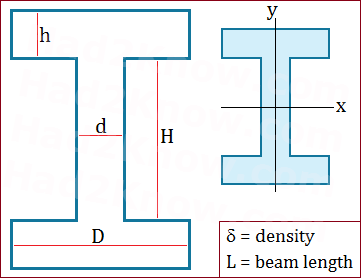# I-Beam Calculator

## Moments and Bending Moments of Inertia, Area, Mass

I- Beam Engineering Calculator
D = cm
d = cm
H = cm
h = cm
L = cm
δ = kg/cm3

Cross-Sectional Area =
cm2
Beam Volume =
cm3
Beam Mass =
kg
Moment of Inertia IX =
kg⋅cm2
Moment of Inertia IY =
kg⋅cm2
Area Moment of Inertia IX =
cm4
Area Moment of Inertia IY =
cm4

I-beams, also called "wide flange" or W-beams, are preferred in construction because their I-shape allows them to withstand strong shearing and bending forces. See also Hollow Rectangular Beams. To calculate loads and forces on an I-beam, you need to know the cross-sectional area, the moments of inertia in the x- and y-directions, as well as the bending moments of inertia (aka area moments of inertia) in the x- and y-directions.Using the image above as a guide, enter the dimensions of the I-beam into the calculator. D is the width of the flange, d is the width of the web (center support column), H is the height of the web, and h is thickness of the flange. In the calculator, L is the total length of the beam and δ is the density of the material.

For the calculator, enter distances in centimeters and the density in kg/cm3. Remember 100 cm = 1 meter and 1000 grams = 1 kg. Use the Had2Know conversion calculator if needed.

### Area, Volume, Mass Equations

The area of an I-beam's cross-section is calculated by adding the areas of the three rectangles that make up the I-shape. The volume is simply area times length. And finally the mass is equal to the density times the volume. The formulas are

Area = Hd + 2hD
Volume = (Hd + 2hD)L
Mass = (Hd + 2hD)Lδ

### Moments of Inertia

Not to be confused with the bending or area moment of inertia below, the moment of inertia quantifies an object's resistance to being spun around an axis. When the axis passes through the centroid in the x-direction or y-direction, the respective equations are

IX = (δ⋅HdL)(H2 + L2)/12 + 2[ (δ⋅hDL)(h2 + L2)/12 + (δ⋅hDL)(H+h)2/4 ]
IY = (δ⋅HdL)(d2 + L2)/12 + 2(δ⋅hDL)(D2 + L2)/12

To calculate the moment of inertia about an axis that is parallel to one of the centroidal axes, use the Parallel Axis Theorem:

IN = IC + mr2

where IN is the new moment of inertia about the line N, IC is a centroidal moment of inertia, m is the mass, and r is the distance between axes.

### Area Moments of Inertia

The area moment of inertia is also known as the bending moment or second moment of inertia. Its units are cm4 and should not be confused with the "regular" moment of inertia above, whose units are kg⋅cm2. Among beams with the same cross-sectional area but different shapes, I-beams have high bending moments in the x-direction, which means they are good at resisting shearing and bending when force is applied to the flanges. The bending moments in the x- and y-directions passing through the center of the I are

IX-area = H3d/12 + 2[ h3D/12 + hD(H+h)2/4 ]
IY-area = d3H/12 + 2[ D3h/12 ]

To find the area moment of inertia with respect to an axis that is parallel to a centroidal axis, there is another version of the Parallel Axis Theorem:

IN-area = IC-area + Ar2

where A is the area of the cross-section.